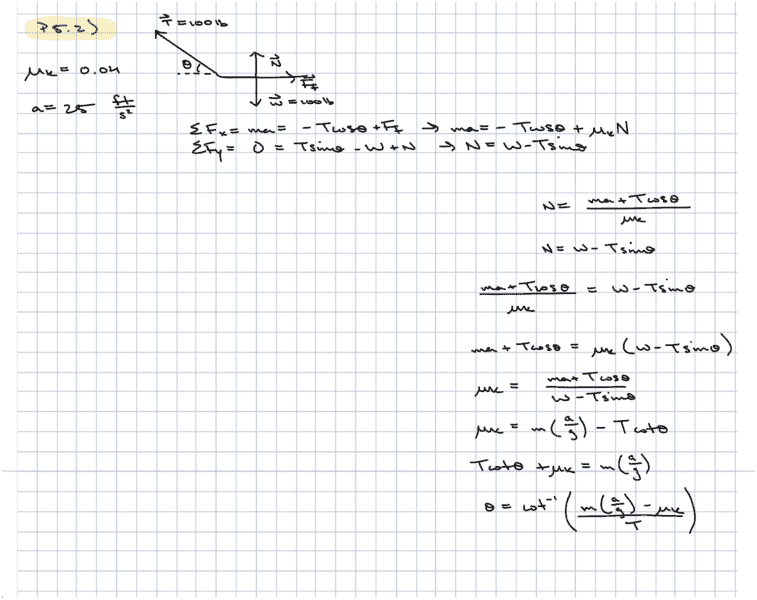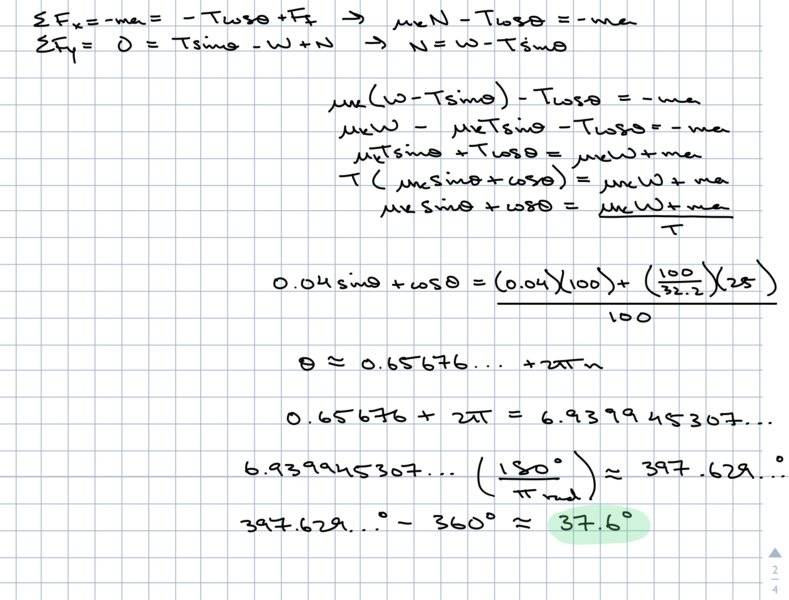# Particle Newtonian Mechanics: a father is pulling a 100 lb sled with a rope at an angle

## Homework Statement

The problem states that there is a father pulling a 100 lb sled with a force of 100 lb at some angle. At what angle does the father have to pull in order for the sled to accelerate at 25 ft/s^2? The coefficient of kinetic friction here is given as 0.04.

F = ma

## The Attempt at a Solution

https://imgur.com/a/UQzd11WMy solution is in the attached image. I did a basic free body diagram and then did a summation of forces in the x and y directions. I solved for N since we do not know it and I tried to isolate for theta, but my answer contradicts the one I see given in the back of the book.

#### Attachments

Last edited by a moderator:

gneill
Mentor
Please UPLOAD any essential images to the PF server (use the UPLOAD button). We find that off-site stored content tends to "evaporate" over time.

verty
Homework Helper

## Homework Statement

The problem states that there is a father pulling a 100 lb sled with a force of 100 lb at some angle. At what angle does the father have to pull in order for the sled to accelerate at 25 ft/s^2? The coefficient of kinetic friction here is given as 0.04.

F = ma

## The Attempt at a Solution

https://imgur.com/a/UQzd11W
My solution is in the attached image. I did a basic free body diagram and then did a summation of forces in the x and y directions. I solved for N since we do not know it and I tried to isolate for theta, but my answer contradicts the one I see given in the back of the book.

From this statement: ##ma + T \cos\theta = \mu_k (w - T \sin\theta)##, one cannot say that ##\mu_k = m({a \over g}) - T \cot\theta##.

EDIT: Sorry, I mixed up two formulas and the formula I pasted here was wrong. Please see post #11.

Last edited:
From this statement: ##ma + T \cos\theta = \mu_k (w - T \sin\theta)##, one cannot say that ##\mu_k = m({a \over g}) - T \cot\theta##.

I know of a way to solve this using the formula: ##a \sin\theta + b \cos\theta = R \cos(\theta + \alpha)## where ##R = \sqrt{a^2 + b^2}## and ##\alpha = \tan^{-1}({b \over a})##.

You would do the following: $$ma + T \cos\theta = \mu_k (w - T \sin\theta) \\ \mu_k T \sin\theta + T \cos\theta = \mu_k w - ma \\ \mu_k \sin\theta + \cos\theta = {\mu_k w - ma \over T}$$
Then use that formula and solve for ##\theta##.
Thanks for the reply. A little lost on where this formula came from, and how to use it? Is there another way?

haruspex
Homework Helper
Gold Member
From this statement: ##ma + T \cos\theta = \mu_k (w - T \sin\theta)##.
That's an unusual way to write it, taking forces as positive right (in the diagram) but acceleration as positive left.
Thanks for the reply. A little lost on where this formula came from, and how to use it? Is there another way?
Do you know how to expand cos(θ+α)?

That's an unusual way to write it, taking forces as positive right (in the diagram) but acceleration as positive left.

Do you know how to expand cos(θ+α)?
Oh I see. I must've made a mistake. I mean to take right as positive and left as negative.
And no, unfortunately, I do not know how to expand that.37.6 degrees is listed as the correct answer. I found a way to obtain it, but it’s of no use since it doesn’t really make sense to me.

I used SymboLab to solve the equation and was hoping there was an algebraic way to do this. Also, SymboLab listed several possibilities, and I had to choose the second option in order to get the answer.
Also, I’m not sure why I had to subtract 360 to get the right answer.

#### Attachments

verty
Homework Helper
That's an unusual way to write it, taking forces as positive right (in the diagram) but acceleration as positive left.

That's a very good point. Making a negative was risky with regard to signs.

gneill
Mentor
And no, unfortunately, I do not know how to expand that.
You should have covered the basic trig identities at some point in your math course.

A way to do this problem without bringing trig functions into it (at least not until the very end when you wish to determine the angle value) is to start by assuming that the tension components are separate variables, say ##T_x## and ##T_y## where ##T = \sqrt{T_x^2 + T_y^2}## is one equation and your equation of motion using these variables is the other. Two equations in two unknowns.

That's a very good point. Making a negative was risky with regard to signs.

Do you mind elaborating on how to solve for theta by hand? That is, using a simple calculator, but not a computer algebra system

verty
Homework Helper
Do you mind elaborating on how to solve for theta by hand? That is, using a simple calculator, but not a computer algebra system

First I must apologise because I was in a rush and I copied the wrong formula when I first responded to this thread. Here is the formula I meant to copy:
$$a \sin\theta + b \cos\theta = \sqrt{a^2 + b^2} \cos(t - \tan^{-1}({a \over b}))$$
I have verified this with a graphical solution to this problem and it is correct. There is also a sin formula which you can see here.

Now, how to solve it by hand. I know of no better way than this:

1. Use the forces in the x direction and the forces in the y direction to get two equations in two variables.
2. Substitute to get one equation and isolate sin and cos on one side.
3. Use the addition formula above (or the sin one) and solve for ##\theta##.

Also, be careful about the units because the numbers won't work if the units are wrong.

verty
Homework Helper
Actually there is a way to do it without needing any fancy formulas. I didn't realise this could be done. I saw it in another thread.

We have two formulas, one with ##T \sin\theta## and one with ##T \cos\theta##. If you write them like this:
1. ##T \sin\theta = ...##
2. ##T \cos\theta = ...##

Then square them and add them:
3. ##T^2 = ...##

Then it's just plain algebra. It's actually very nice and I prefer this to using those addition formulas. There you go, @rugerts.

Actually there is a way to do it without needing any fancy formulas. I didn't realise this could be done. I saw it in another thread.

We have two formulas, one with ##T \sin\theta## and one with ##T \cos\theta##. If you write them like this:
1. ##T \sin\theta = ...##
2. ##T \cos\theta = ...##

Then square them and add them:
3. ##T^2 = ...##

Then it's just plain algebra. It's actually very nice and I prefer this to using those addition formulas. There you go, @rugerts.
Thank you so much for your help! I still cannot seem to get it, but it's probably due to some poor algebra. Thanks anyways

hutchphd
Homework Helper
Thank you so much for your help! I still cannot seem to get it, but it's probably due to some poor algebra. Thanks anyways
Being terrible at algebra myself, I will give you advice I have seen ignored by far too many students at their peril:
Every time you manipulate an equation check that each term still has the appropriate units. You will catch (and would have caught in the above) almost all the "stupid" algebra mistakes. Make it a habit.....!

Being terrible at algebra myself, I will give you advice I have seen ignored by far too many students at their peril:
Every time you manipulate an equation check that each term still has the appropriate units. You will catch (and would have caught in the above) almost all the "stupid" algebra mistakes. Make it a habit.....!
This is good advice. Thank you.

neilparker62
Homework Helper
Do you mind elaborating on how to solve for theta by hand? That is, using a simple calculator, but not a computer algebra system
You can use 't' substitutions for cos(θ) and sin(θ) where 't' = tan(θ/2). Then:

$$cos(θ)=\frac{1-t^2}{1+t^2}$$ and $$sin(θ)=\frac{2t}{1+t^2}$$

You will end up with a quadratic in t.